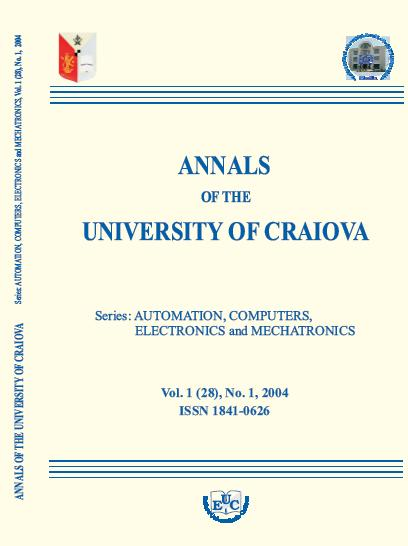# ANNALS OF THE UNIVERSITY OF CRAIOVA

## Series: AUTOMATION, COMPUTERS, ELECTRONICS and MECHATRONICS ISSN: 1841-0626Home Current Issue Archive Editorial Board Instruction for authors Search Contact Us Year 2004 Volume 1(28) no. 1 CONTINUOUS-TO-DISCRETE MODEL CONVERSION OF A SYSTEM WITH STATE DELAY Bujgoi Gheorghe Abstract: In practical applications, a very short sampling period interval will lead to an increase in the computation time and will create a serious dimensionality problem. Based on the model derived in this paper, we can utilize a longer sampling time for sampled-data models. Model conversion of a state-delay system is presented. The discrete time model is derived from the continuous-time system, that it has a wider range for the sampling period in real implementation. A simple method is also proposed to determine the unknown state-delay time from the available discrete-time model. Keywords: state delay, state-space model, model conversion EM ALGORITHM PROBABILITY ESTIMATION IN DESIGN OF MULTI-MODEL PREDICTIVE CONTROLLER Filasová Anna, Sás Martin Abstract: The paper presents model-based predictive controller design based on a mixture distribution. The control sequence derivation is based on the optimization criterion and its minimalization on the prediction horizont. The on-line estimation of parallel models probabilities is achieved. The paper confronts effectiveness of two approaches to probability estimation (EM algorithm and bayesian approach), with given mathematical solution for EM algorithm. The algorithm is illustrated in simulation enviroment MATLAB using the angular positioning system model. Keywords: Model-based predictive control, mixture distribution, EM algorithm, adaptive control, probability estimation. TRUETIME LIBRARY FOR SIMULATING SIMPLE REAL-TIME CONTROL SYSTEMS Cosmin Ionete Abstract: This article is focused in introducing a new MATLAB/Simulink-based tool for simulation of real-time distributed control systems. This introduction is made by examples, to facilitate the understanding of main skills of the TrueTime tool. After a short presentation, we introduce the Real Time Kernel by the use of a PID control law implementation. We shall emphasize the differences between the classical simulation and real-time simulation. Also is presented the influence o real-time parameters on the real control system. The flexibility of the TrueTime Simulink Library extension is underlined by using both MATLAB and Simulink implementation of the PID control law. Keywords: Real time control systems, Event based simulation, Shared resources, Real Time kernel, Feedback scheduling, Networked control systems. THE SIMULATION FOR THE TURBINE  GENERATOR GROUP Camelia Maican Abstract: One of the essential conditions, imposed by the coupling to an energetically area of the turbo-generators, is given by limits of variation of the electrical generators frequency forced for the National Energetically Power System at ±0.2 Hz, respectively ± 0.4%. In this paper we study power and frequency regulation of the turbo generators couple to power area. This simulations were made using the Matlab Simulink libraries. Keywords: power area, steam turbine, electrical generator. SOME NEW RESULTS OF STABILITY OF SYSTEMS WITH SATURATION AND DELAY IN COMMAND AND DELAY IN STATE Marcel Nicola Abstract: In this section we consider systems with delay in state and command and saturation in command, and using a transformation given in ( Zheng, 2001) the initial system is transformed in one whithout dealy but which contain saturation in command. The investigations are continuing using some results from the study of systems with saturation in command (Lee and Hedrick 1995). In this manner, using the transformation relation between the state of the initial system with delay and the state of the transformated system whitout delay, we can formulate some results regarding the stabilization of the initial system with delay and saturation in command and delay in state. The Propozitions 1-6 from this paper are personal results of the author. Keywords: delay in command and state, stabilization, saturation in command APPROXIMATION OF THE DYNAMICAL MODEL OF NONLINEAR DISTRIBUTED PARAMETER BIOREACTORS VIA ORTHOGONAL COLLOCATION Emil Petre Abstract: In this paper the dynamics of a class of propagation bioprocesses that are carried out in fixed bed reactors without dispersion is analysed. Since the dynamics of these processes are described by partial differential equations either for simulation or for control of these processes one method consists of approximation of their infinitely order associated models by finite order models. These approximate models consist of a set of ordinary differential equations obtained here by orthogonal collocation method. Since it is difficult to know the connections between the original distributed parameter model and its approximate version, our objective is to analyse the behaviour of both models to view their dynamical properties. Computer simulations conducted in the case of a fixed bed reactor are included to illustrate the dynamical behaviour of the two classes of models. Keywords: Nonlinear systems, Bioprocesses, Distributed parameter systems, Orthogonal collocation, Fixed bed reactor. SOME ASPECTS REGARDING NUMERICAL SIMULATION OF CHAOTIC ATTRACTORS Dan Selisteanu, Dan Popescu Abstract: This work presents some considerations regarding the numerical simulation of chaotic attractors. The paper analyses some theoretical aspects of the chaotic behaviour of the dynamical systems. Numerical simulation of chaotic attractors using simulation packages like MATLAB and PHASER is examined. In order to compare the simulation results, illustrative examples such as Lorenz or Rössler strange attractors are considered. Keywords: Chaos, Attractors, Numerical simulation